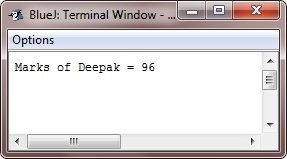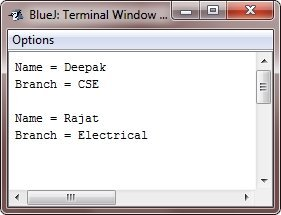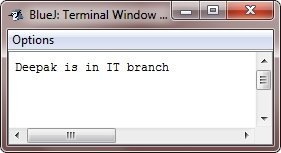# Java Variable Types

Variable is basically a name of the memory location. Based on the data type of variable, memory layout and size can be different.

Here the following table lists all the three types of variables available in Java along with their meaning:

Variable Type Meaning
Local Variables Local variables Java, are created locally, such as inside the method, constructor, or any block and these local variables automatically destroyed upon the exits from that method, constructor, or any block where they are created
Instance Variables Instance variables in Java, are created whenever an object is created through the use of new operator. You can create instance variables in Java, in a class, but outside any block, method, or any constructor.
Static Variables Static variables are also called as class variables, are created through the use of static keyword in a class, but outside any block, method, or any constructor

## Local variables in Java

Here is an example program, demonstrates the concept and use of local variables in Java. Here marks is the local variable.

```/* Java Variable Types - Java Local Variables - Example Program */

public class JavaProgram
{
void printMarks()
{
int marks = 0;
marks = marks + 96;
System.out.println("Marks of Deepak = " + marks);
}
public static void main(String[] args)
{
JavaProgram jp = new JavaProgram();
jp.printMarks();
}
}```

Here is the sample output produced by the above Java program:## Instance Variables in Java

Here is an example program, illustrates the concept and use of instance variables in Java:

```/* Java Variable Types - Java Instance Variables - Example Program */

import java.io.*;

public class JavaProgram
{
public String studname; // this instance variable is visible for any child class
private String studbranch;  // this instance variable is only visible to the JavaProgram class

public JavaProgram(String name)
{
studname = name;
}
public void initializeBranch(String branch)
{
studbranch = branch;
}
public void printStudent()
{
System.out.println("Name = " + studname);
System.out.println("Branch = " + studbranch + "\n");
}

public static void main(String args[])
{
JavaProgram jp1 = new JavaProgram("Deepak");
jp1.initializeBranch("CSE");
jp1.printStudent();
JavaProgram jp2 = new JavaProgram("Rajat");
jp2.initializeBranch("Electrical");
jp2.printStudent();
}
}```

Here is the sample output produced by this Java Program:## Static Variables in Java

Here is an example program, helps you in understanding the static variables in Java:

```/* Java Variable Types - Java Static Variables - Example Program */

import java.io.*;

public class JavaProgram
{
private static String studname; // this is a private, static variable
public static final String studbranch = "IT";   // constant variable

public static void main(String args[])
{
studname = "Deepak";
System.out.println(studname + " is in " + studbranch + " branch");
}
}```

The above Java program will produce the following output:Tools
Calculator